## 在 PID 调节器中分析设计

### 绘制系统响应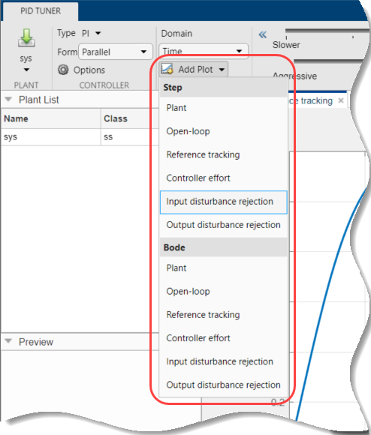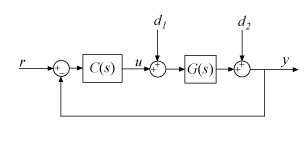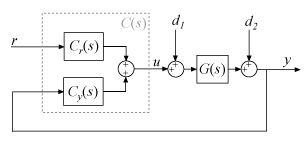$\frac{GC}{1+GC}$（从 r 到 y）

$\frac{G{C}_{r}}{1-G{C}_{y}}$（从 r 到 y）

$\frac{C}{1+GC}$（从 r 到 u）

$\frac{{C}_{r}}{1-G{C}_{y}}$（从 r 到 u）

$\frac{G}{1+GC}$（从 d1 到 y）

$\frac{G}{1-G{C}_{y}}$（从 d1 到 y）

$\frac{1}{1+GC}$（从 d2 到 y）

$\frac{1}{1-G{C}_{y}}$（从 d2 到 y）

#### 将调节后响应与基线响应进行比较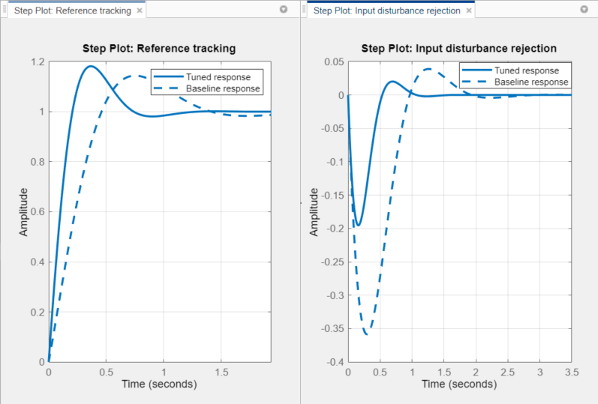• 使用语法 `pidTuner(sys,C0)` 在打开 PID 调节器时加载基线控制器。

• 通过点击导出箭头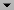并选择另存为基线，可立即将当前 PID 调节器设计作为基线控制器。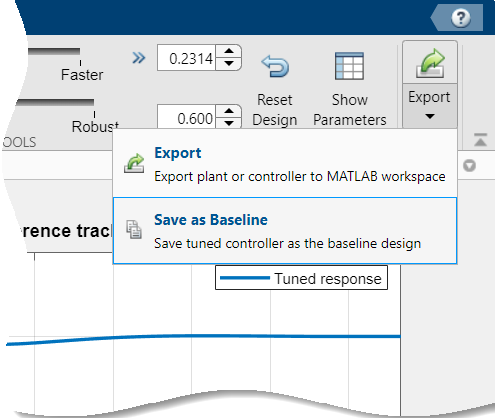执行此操作时，当前的调节后响应将成为基线响应。对当前设计的进一步调整会创建新的调节后响应线。

要隐藏基线响应，请点击选项，并清除显示基线控制器数据

### 查看系统特性值

• 直接在响应图上 - 使用右键点击菜单添加特性，显示为蓝色标记。然后，左键点击标记以显示对应的数据面板。

• 性能和稳健性表中 - 要显示此表，请点击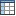显示参数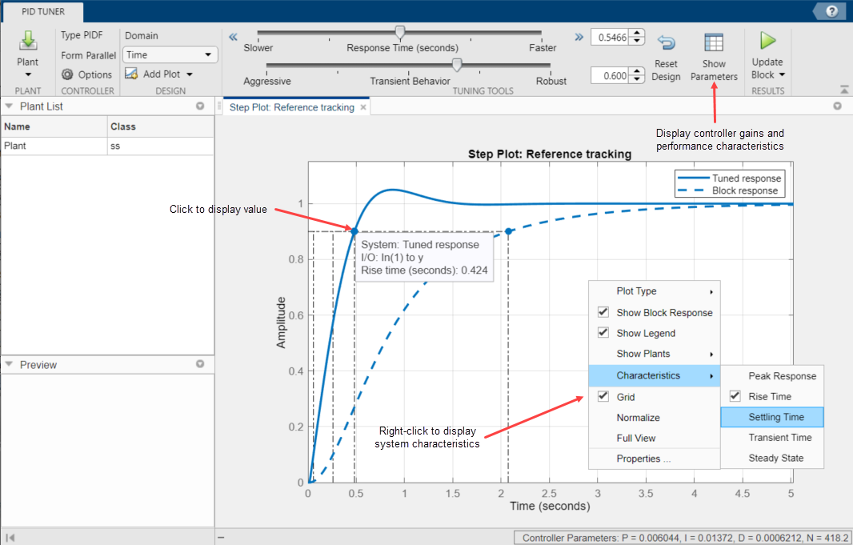### 细化设计

• 时间（默认值）- 使用响应时间滑块来加快或减慢控制系统的闭环响应速度。使用瞬态特性滑块，使控制器更积极地抑制扰动或更稳健地应对被控对象的不确定性。

• 频率 - 使用带宽滑块来加快或减慢控制系统的闭环响应速度（响应时间为 2/wc，其中 wc 是带宽）。使用相位裕度滑块，使控制器更积极地抑制扰动或更稳健地应对被控对象的不确定性。